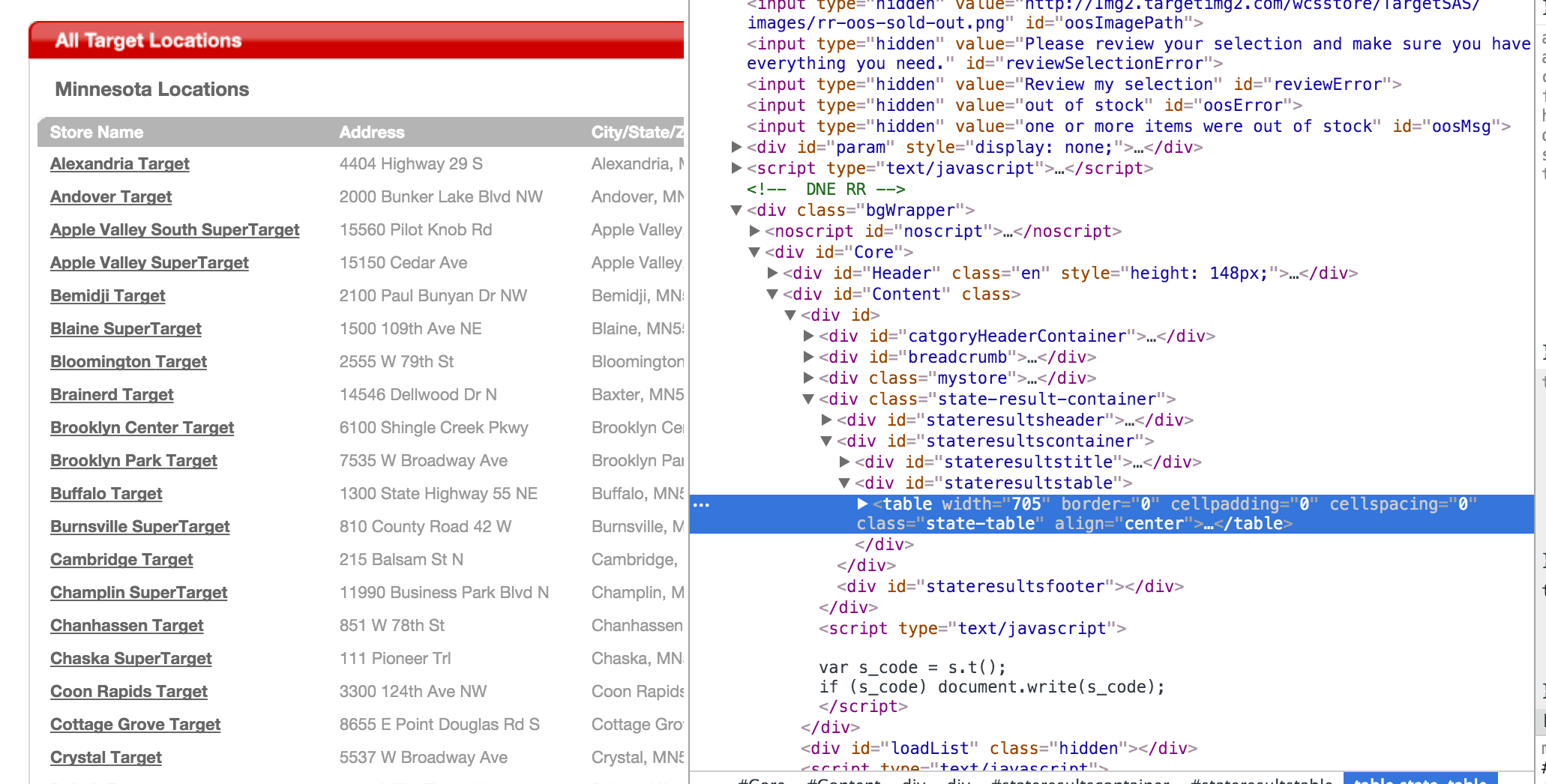Want to share your content on R-bloggers? click here if you have a blog, or here if you don't.

I just finished developing a presentation for Target Analytics Network showcasing geospatial and mapping tools in R . I decided to use Target store locations as part of a case study in the presentation. The problem: I didn’t have any store location data, so I needed to get it from somewhere off the web. Since there some great tools in R to get this information, mainly `rvest` for scraping and `ggmap` for geocoding, it wasn’t a problem. Instead of just doing the work, I thought I should share what this process looks like:

First, we can go to the target website and find stores broken down by state.After finding this information, we can use the `rvest` package to scrape the information. The URL is so nicely formatted that you can easily grab any state if you know the state’s mailing code.

```# Set the URL to borrow the data.
TargetURL <- paste0('http://www.target.com/store-locator/state-result?stateCode=', state)
```

Now we can set a state — Minnesota’s mailing code is MN.

```# Set the state.
state <- 'MN'
```

Now that we have the URL, let’s grab the html from the webpage.

```# Download the webpage.
TargetWebpage <-
TargetURL %>%
```

Now we have to find the location of the table in the html code.Once we have found the html table, there are a number of ways we could extract from this location. I like to copy the the XPath location. It’s a bit lazy, but for the purpose of this exercise it makes life easy.

Once we have the XPath location, it’s easy to exact the table from the Target’s webpage. First we can pipe the html through the `html_nodes` function, this will isolate the html responsible for creating the store locations table. After that we can use the `html_table` to parse the html table into an R list. Let’s then use the `data.frame` function to take the list to a data frame and use the `select` function from the `dplyr` library to select specific variables. The problem with extracting the data is that the city, state, and zip code are in one column. Well its not really a problem for this exercise, but its maybe the perfectionist in me. Let’s use the `separate` function in the `tidyr` library to make city, state, and zipcode their own columns.

```# Get all of the store locations.
TargetStores <-
TargetWebpage %>%
rvest::html_nodes(xpath = '//*[@id="stateresultstable"]/table') %>%
rvest::html_table() %>%
data.frame() %>%
dplyr::select(`Store Name` = Store.Name, Address, `City/State/ZIP` = City.State.ZIP) %>%
tidyr::separate(`City/State/ZIP`, into = c('City', 'Zipcode'), sep = paste0(', ', state)) %>%
dplyr::mutate(State = state) %>%
dplyr::as_data_frame()
```

Let’s get the coordinates for these stores; we can pass each store’s address through the `geocode` function which obtains the information from the Google Maps API — you can only geocode up to 2500 locations per day for free using the Google API.

```# Geocode each store
TargetStores %<>%
dplyr::bind_cols(
ggmap::geocode(
paste0(
TargetStores\$`Store Name`, ', ',
TargetStores\$City, ', ',
TargetStores\$State, ', ',
TargetStores\$Zipcode
),
output = 'latlon',
)
)
```

Now that we have the data, let’s plot. In order to plot this data, we need to put it in a spatial data frame — we can do this using the `SpatialPointsDataFrame` and `CRS` functions from the `sp` package. We need to specify the coordinates, the underlying data, and the projections

```# Make a spatial data frame
TargetStores <-
sp::SpatialPointsDataFrame(
coords = TargetStores %>% dplyr::select(lon, lat) %>% data.frame,
data = TargetStores %>% data.frame,
proj4string = sp::CRS("+proj=longlat +datum=WGS84 +ellps=WGS84 +towgs84=0,0,0")
)
```

Now that we have a spatial data frame, we can plot these points — I’m going to plot some other spatial data frames to make add context for the Target store point data.

```# Plot Target in Minnesota
plot(mnCounties, col = '#EAF6AE', lwd = .4, border = '#BEBF92', bg = '#F5FBDA')
plot(mnRivers, lwd = .6, add = TRUE, col = '#13BACC')
plot(mnLakes, border = '#13BACC', lwd = .2, col = '#EAF6F9', add = TRUE)
plot(TargetStores, add = TRUE, col = scales::alpha('#E51836', .8), pch = 20, cex = .6)
```Yes! We’ve done it. We’ve plotted Target stores in Minnesota. That’s cool and all, but really we haven’t done much with the data we just obtained. Stay tuned for the next post to see what else we can do with this data.

UPDATE: David Radcliffe of the Twin Cities R User group presented something similar using Walmart stores.